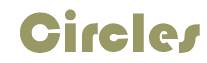A circle is a set of points equal distance from a single center point.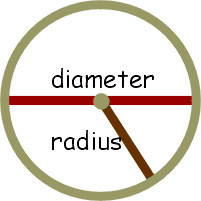The distance around the circle is known as the Circumference. The ratio of the circumference and the diameter create the number known as pi.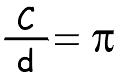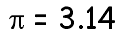The distance from one side of the circle through the middle is known as the diameter. The distance from the center to the side of the circle is known as the radius.

The radius is one half of the diameter.
 Find the diameter using this formula. Find the radius using this formula.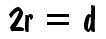If r = 3 then d = 6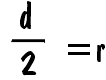If d = 45 then r = 22.5

The circumference of a circle can be found
using either of these formulas.If d = 2 then C = 2(3.14) = 6.28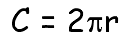If r = 10 then C = 2(10)(3.14) = 62.8
 The area of a circle can be found using this formula.If r = 3 then a = 9(3.14) = 28.26

practice